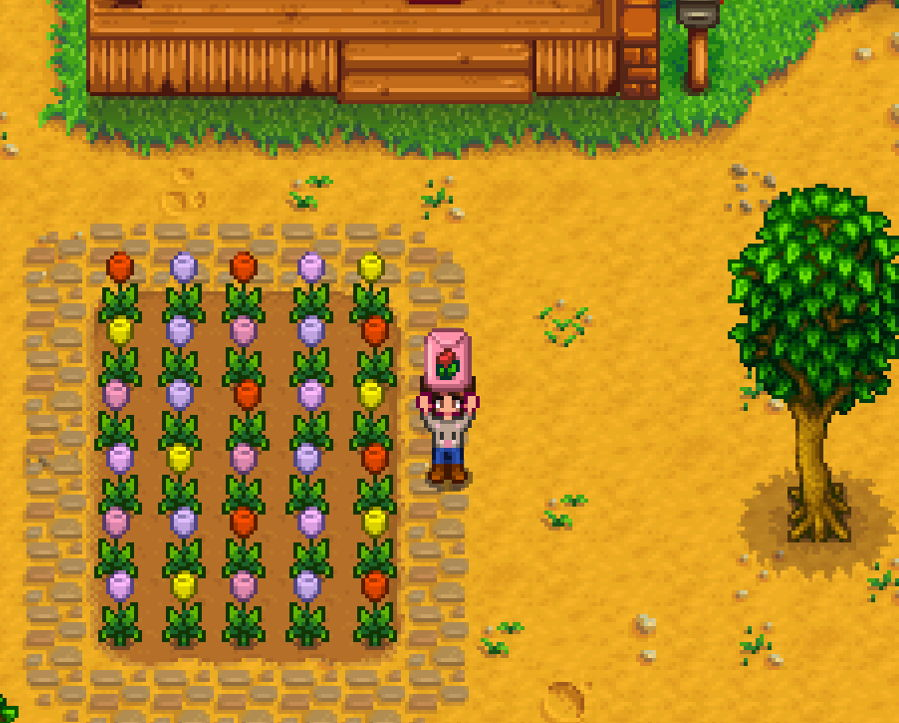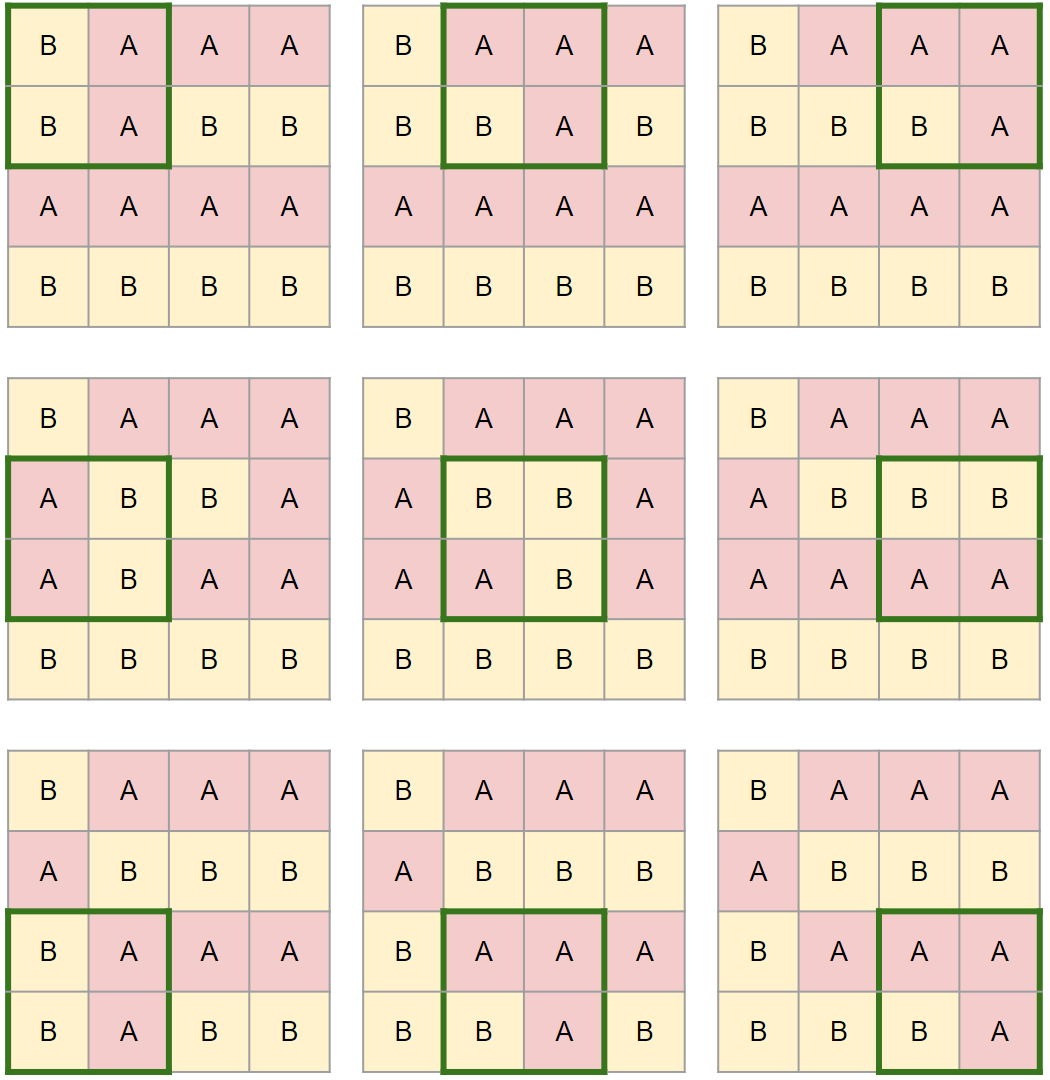시간 제한메모리 제한제출정답맞힌 사람정답 비율
3 초 (추가 시간 없음) 1024 MB19171487.500%

## 문제

Joon-Pyo decorated a flowerbed in front of his home. The flowerbed is in the shape of an $n \times m$ grid, and one flower is planted in each cell. There are 26 colors, one corresponding to each uppercase letter from A to Z. Suddenly, he wanted to redecorate the flowerbed.The flowerbed is too large to adjust the flowers one by one. He rented some equipment that can lift and rotate a square plot of land with a side length of $d$. He planned the construction in the following order, expecting the flowerbed to be properly redecorated.

1. Place the equipment so that exactly the flowers in the first $d$ rows and the first $d$ columns are inside.
2. Rotate the $d \times d$ square inside the equipment $90^\circ$ clockwise. If this square contains flowers from the last $d$ rows and the last $d$ columns, then the construction is finished. Otherwise, if this square does not contain flowers in the last $d$ columns, move the equipment $x$ squares to the right. Otherwise, move the equipment down by $y$ squares and all the way to the left so it contains flowers from the first $d$ columns.
3. Repeat step 2 until construction is finished.

Note that the equipment will never go out of the flowerbed, as $x$, $y$, and $d$ are carefully determined before construction begins.

He cannot start construction without knowing the outcome. Write a program that outputs the result.

## 입력

On the first line, five integers $n$, $m$, $y$, $x$, and $d$ are given. ($1 \leq n \times m \leq 10^6$, $1 \leq y \leq n$, $1 \leq x \leq m$, $1 \leq d \leq \min(n, m)$, $\boldsymbol{n \equiv d \pmod y}$, $\boldsymbol{m \equiv d \pmod x}$).

Each of the next $n$ lines contains exactly $m$ uppercase letters, the current flowerbed.

## 출력

Output $n$ lines, each containing $m$ uppercase letters, the flowerbed after the planned construction.

## 예제 입력 1

4 4 1 1 2
AAAA
BBBB
AAAA
BBBB


## 예제 출력 1

BAAA
ABBB
BAAA
BBBA


## 예제 입력 2

6 5 1 2 3
RBRCY
YBPBR
PBRCY
CYPBR
PBRCY
CYPBR


## 예제 출력 2

PYRBR
CRCBB
PPBPY
CRCYB
YRBCY
PYRBR


## 노트

In the first example, the flowerbed changes as follows: